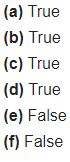# Say True or False: (a) Each angle of a rectangle is a right angle

Say True or False:
(a) Each angle of a rectangle is a right angle.
(b) The opposite sides of a rectangle are equal in length.
( c) The diagonals of a square are perpendicular to length.
(d) All the sides of rhombus are of equal length.
(e) All the sides of a parallelogram are of equal length.
(f) The opposite sides of a trapezium are parallel.